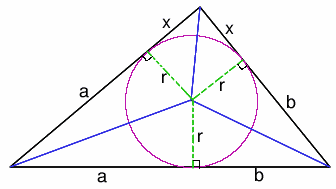#### You may also like### Degree Ceremony

What does Pythagoras' Theorem tell you about these angles: 90Â°, (45+x)Â° and (45-x)Â° in a triangle?### Logosquares

Ten squares form regular rings either with adjacent or opposite vertices touching. Calculate the inner and outer radii of the rings that surround the squares.### Ball Bearings

If a is the radius of the axle, b the radius of each ball-bearing, and c the radius of the hub, why does the number of ball bearings n determine the ratio c/a? Find a formula for c/a in terms of n.

# So Big

##### Age 16 to 18Challenge Level

Eduardo from the British School, Manila, Tomas from Malmesbury School and Philip used the tangent formula and Alex and also Sue Liu of Madras College, St Andrew's sent a solution to this problem which depends on the use of Heron's Formula for the area of a triangle. Well done all of you.FIRST METHOD
Using the tangents of the angles at the centre of the circle and the formula

$\tan X= -\tan(A + B) =\frac{\tan A + \tan B} {\tan A \tan B - 1}$ where $A + B + X = 180^o$,

## $\frac{x}{r}= \frac{\frac{a}{r} + \frac{b}{r}}{\frac{ab}{r^2}-1} = \frac{r(a + b)}{ab - r^2}.$

The area of the triangle is
$(a + b + x)r = (a + b)r + \frac{r^3(a + b)}{ab - r^2}= \frac{(a + b)abr}{ab - r^2}$ as required.

SECOND METHOD
This method uses Heron's formula. The lines from the centre of the circle to the edges each meet the tangents to the circle forming right angles as shown. The triangle has been rotated/reflected so that the side with length (a+b) is the base. (However it is rotated it is the same shape of unknown angles and lengths). As the tangents to a circle from an external point are equal in length (easily proved using congruent triangles) the other lengths of the sides of the triangle can be found. The incircle divides the sides of the triangle into lengths $c' = a + b$, $b' = b + x$ and $a' = x + a$ as shown in the diagram.

The semi-perimeter of the triangle is given by $s = x + a + b$ and from Heron's formula the area of the triangle is

$A = \sqrt{(s(s - a')(s - b')(s - c'))}$

$= \sqrt{((a + b + x)abx)}$

Also, the triangle is divided into three smaller triangles and the total area is given by

$A = \frac{1}{2}(a + b)r + \frac{1}{2}(b + x)r + \frac{1}{2}(x + a)r$

$=(a + b + x)r$.

$\sqrt{abx(a + b + x)} = (a + b + x)r$

$abx(a + b + x) = (a + b + x)^2 r^2$

$abx = (a + b + x)r^2$

$x = \frac{(a + b)r^2}{ (ab - r^2).}$

Hence

$A = (a + b + x)r$

$= \frac{r((a + b)(ab - r^2) + (a + b)r^2)}{ ab - r^2}$

$= \frac{abr(a + b)}{ab - r^2.}$

An alternative method, not using Heron's formula, is based on finding $x$ in terms of $a$ and $b$ using the tangents of the angles at the centre of the circle.